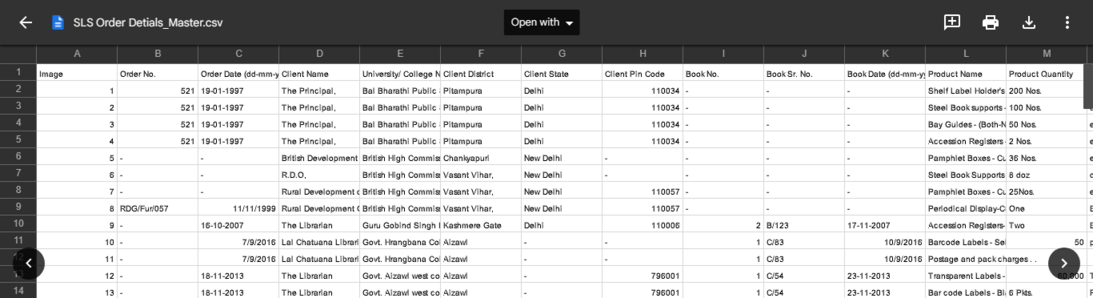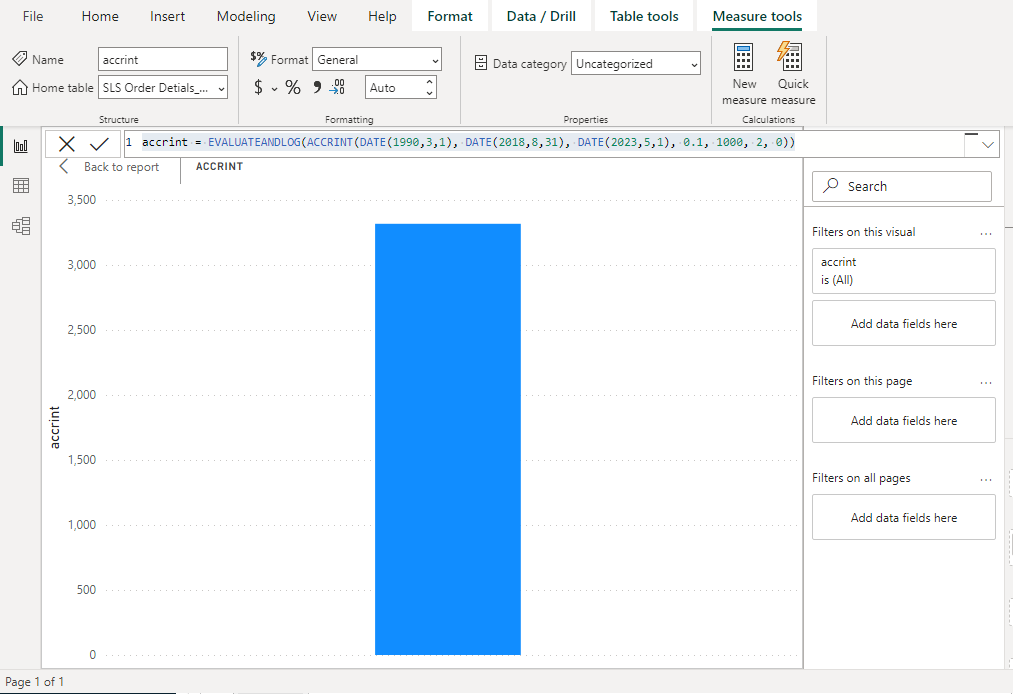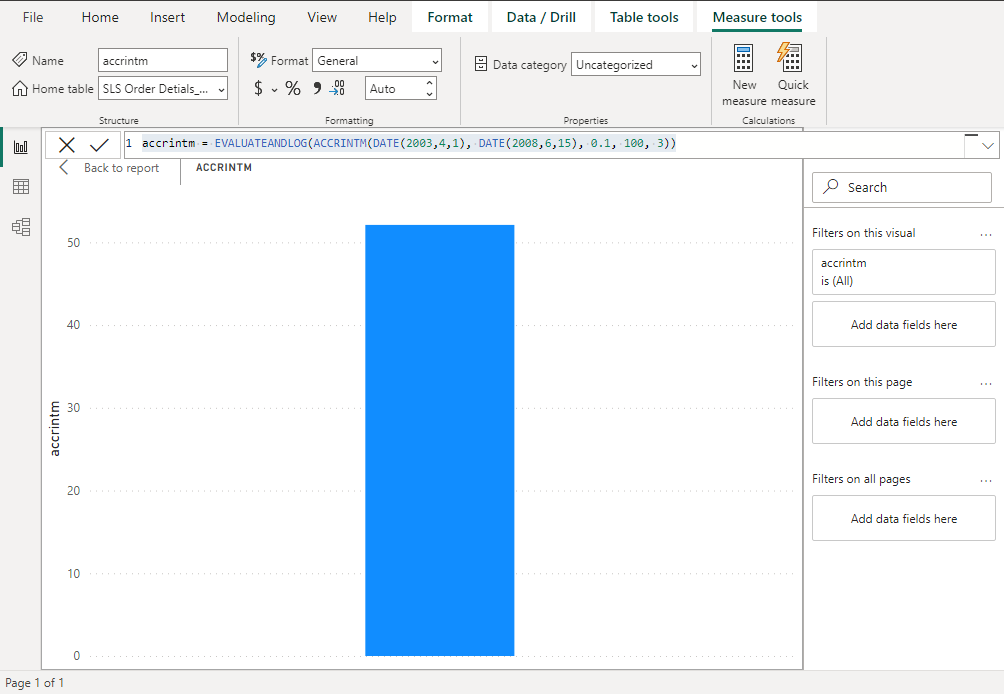Open in App
Not now

# Power BI – DAX Accrued Interest function

• Last Updated : 30 Jan, 2023

Interest that has accrued on a loan over time but has not yet been paid or collected by the borrower or lender is referred to as accrued interest. Regardless of when it will be paid, accrued interest is the total amount of interest that has been incurred or earned during a reporting period under accrual-based accounting. The lender’s interest revenue and receivable account, or the borrower’s interest expense and payable account, make up the adjusting entry for accumulated interest. Bond accrued interest is interest that has accrued since the bond’s last interest payment day but has not yet been paid.

## Accrued Interest Financial Functions in Power BI

Financial functions in DAX are used in formulas that do financial calculations, such as net present value and rate of return. These capabilities are analogous to the financial capabilities of Microsoft Excel. Here, we are applying the functions on a sample library supplies dataset. A screenshot of the same can be seen below:### DAX ACCRINT Financial Function

It gives back any accrued interest on security that produces periodic interest.

Syntax: ACCRINT(<issue>, <first_interest>, <settlement>, <rate>, <par>, <frequency>[, <basis>[, <calc_method>]])

### Parameters

Return Value

The accrued interest.

Notes to be considered:

In order to be used in calculations, dates are recorded as consecutive serial numbers. Since January 1, 2008, is 39,448 days after December 30, 1899, it is represented in the DAX as day 0, and December 30, 1899, as day 0. Calculating ACCRINT is done as follows:Where,

• Ai = number of days that have been accumulated for the ith quasi-coupon period within an odd term.
• NC = number of quasi-coupon periods that are compatible with odd periods. If there is a fraction in this number, round it up to the nearest full number.
• NLi = The quasi-coupon period’s typical length in days inside the odd period.

Numeric values are used for the issue, first interest, and settlement. The nearest integer is used to round the frequency and basis. A failure is indicated if, The dates issue, first interest, or settlement are invalid.

issue ≥ settlement.

rate ≤ 0.

par ≤ 0.

Frequency is any number other than 1, 2, or 4. The rate is 0. Par is 0. The basis is 0 or the Basis is more than 4. In calculated columns or with RLS rules, this function is not supported for use in Direct Query mode.

Example: accrint = EVALUATEANDLOG(ACCRINT(DATE(1990,3,1), DATE(2018,8,31), DATE(2023,5,1), 0.1, 1000, 2, 0))Returns the interest that has accumulated from issuance to settlement on security with the aforementioned terms.

### DAX ACCRINTM Financial Function

This function returns the interest that has accrued on a security that will mature with interest.

Syntax: ACCRINTM(<issue>, <maturity>, <rate>, <par>[, <basis>])

Return Value

The accrued interest.

Notes to be considered:

In order to be used in calculations, dates are recorded as consecutive serial numbers. Since January 1, 2008, is 39,448 days after December 30, 1899, it is represented in the DAX as day 0, and December 30, 1899, as day 0. The formula for ACCRINTM is as follows:Where,

• A- number of days accumulated that are counted on a monthly basis. The number of days between the issue date and the maturity date is used for interest at maturity items.
• D- Annual Year Basis.

The values for issue and maturity are reduced to integers. The base is rounded up to the next whole number. A failure is indicated if, Issue or maturity dates are invalid.

problem: maturity.

par 0. basis 0 or basis > 4. rate 0.

In calculated columns or with RLS rules, this function is not supported for use in Direct Query mode.

Example: accrintm = EVALUATEANDLOG(ACCRINTM(DATE(2003,4,1), DATE(2008,6,15), 0.1, 100, 3))Returns the accumulated interest for security in accordance with the aforementioned terms.

My Personal Notes arrow_drop_up